## Transfer Function of Terminated Two Port Network:

A ratio of voltage or current at one port to the voltage or current at the other port is called a Transfer Function of Terminated Two Port Network. In the last sections, we have studied how to obtain two port network parameters. In this section, we will study how to determine driving point and transfer functions of a two port by use of two-port parameters.

First consider that the two port network is not terminated in source and load impedances. Let us express the transfer functions in terms of z and y parameters. Consider z parameter equations for a two port network.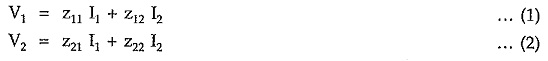When port-2 is open circuited, I2 = 0. Then we can write equations (1) and (2) respectively as,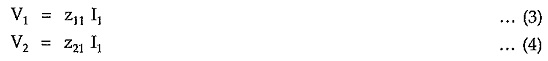From equation (4), we can express current I1 interms of voltage V2 as,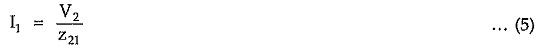Putting value of I1 in equation (3), we can write,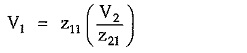Hence, open circuit voltage ratio of two port network is given by,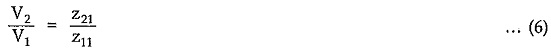Now consider y-parameter equations for a two port network.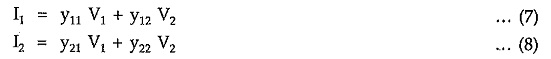When port 2 is open circuited, I2 = 0. Then equation (8) can be written as,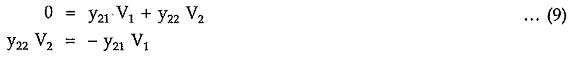Hence, open circuit voltage ratio of two port network interms of y-parameters is given by,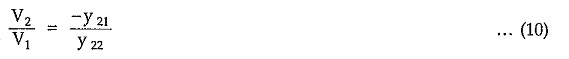We can also determine short circuit current ratio of a two port network in terms of z and y parameters with condition that V2 = 0.

When port 2 is short circuited, V2 = 0. Then equation (2) can be written as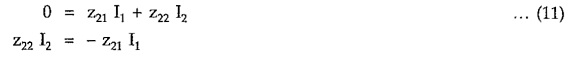Hence short circuit current ratio of a two port network interms of z parameters is given by,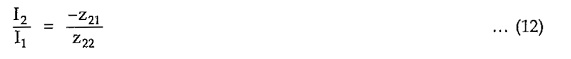Similarly equations (7) and (8) respectively can be written as,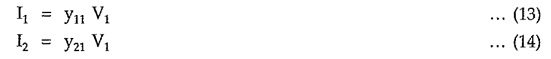From equation (14), we can express voltage V1 interms of current I2 as,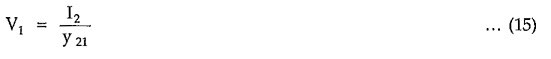Putting value of V1 in equation (13), we can write,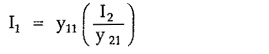Hence, short circuit current ratio of a two port network interms of y-parameters is given by,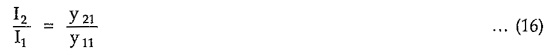Now consider a two port network expressed in terms of z parameters, terminated in impedance ZL as shown in the Fig. 6.18.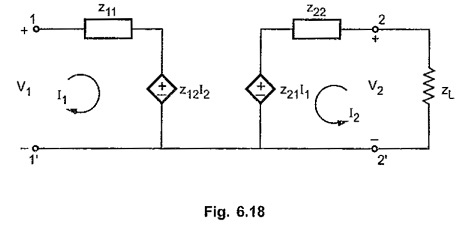From equations of z parameters we can write,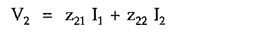But from the network it is clear that, V2 = – I2 ZL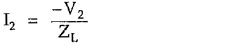Putting value of I2 in above equation,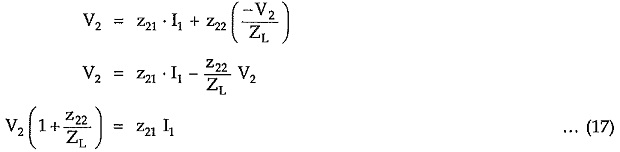The transfer impedance Z21 can be obtained from above equation as,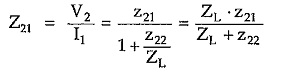Putting value of I1 in equation for V1 as follows,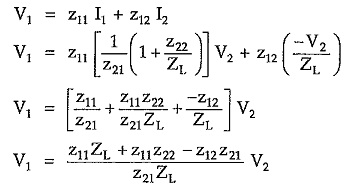The voltage ratio of transfer function of terminated two port network is given by,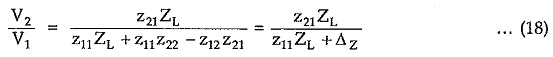Putting value of V2 in equation for V2, for we get,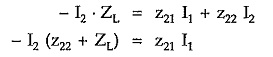The current ratio transfer function for terminated network is given by,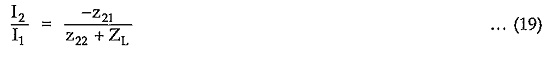The driving point impedance of a terminated two port network can be obtained from equations (17) and (18) as follows,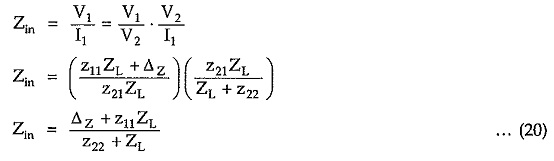Consider a two port network terminated at both the ports of a network as shown in the Fig. 6.19.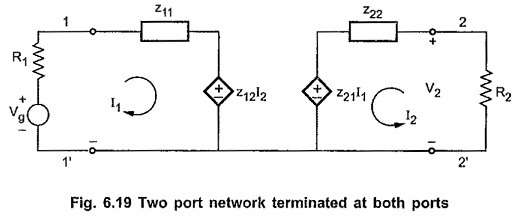Applying KVL at input side, we can write,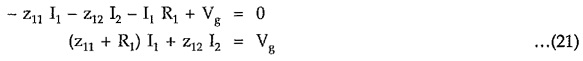Applying KVL at output side, we can write,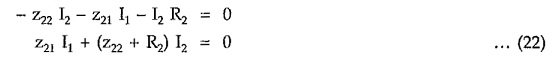But V2 = -I2 R2

Putting values in equation (22), we get,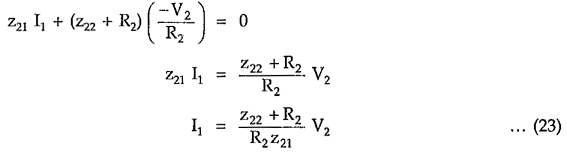Putting value of I1 in equation (21) and writing equation in terms of voltages Vg and V2, we get,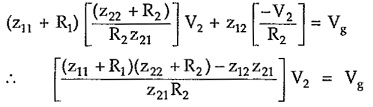Hence, the voltage ratio transfer function of terminated two port network terminated at both the ports is given by,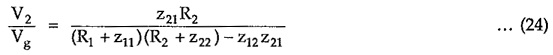Scroll to Top